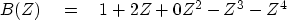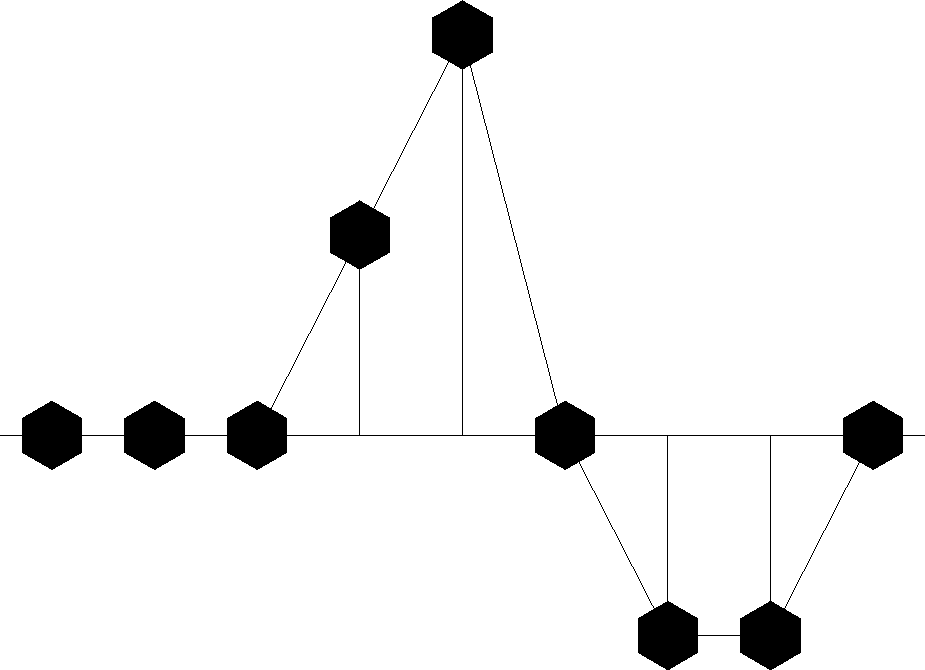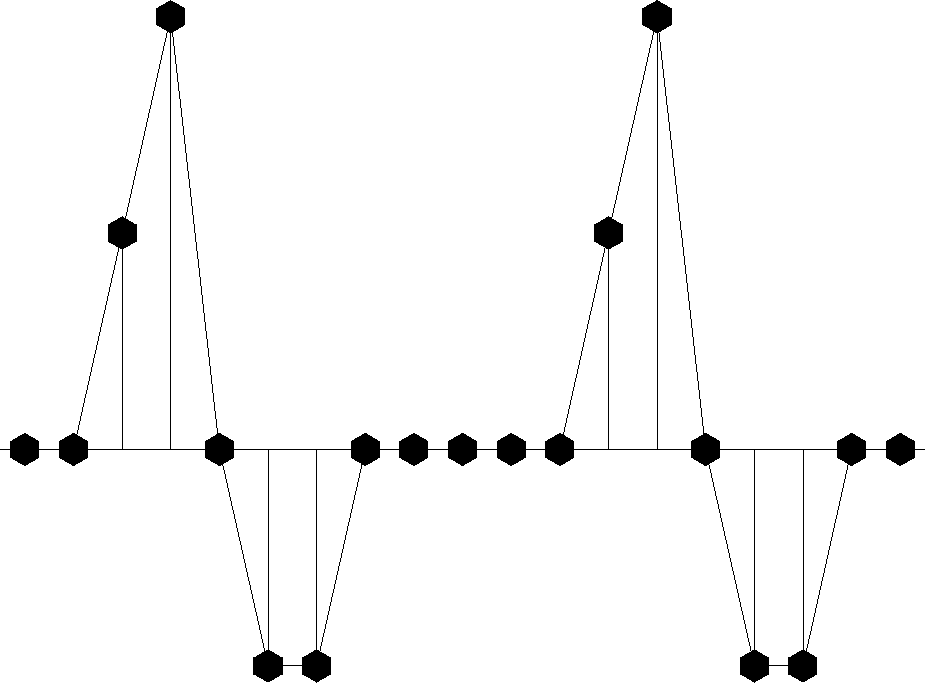Next: Linear superposition Up: Convolution and Spectra Previous: Convolution and Spectra

# SAMPLED DATA AND Z-TRANSFORMS

Consider the idealized and simplified signal in Figure 1.

 triv1 Figure 1 A continuous signal sampled at uniform time intervals. (Press button for trivial interaction with plot.)To analyze such an observed signal in a computer, it is necessary to approximate it in some way by a list of numbers. The usual way to do this is to evaluate or observe b(t) at a uniform spacing of points in time, call this discretized signal bt. For Figure 1, such a discrete approximation to the continuous function could be denoted by the vector(1)
Naturally, if time points were closer together, the approximation would be more accurate. What we have done, then, is represent a signal by an abstract n-dimensional vector.

Another way to represent a signal is as a polynomial, where the coefficients of the polynomial represent the value of bt at successive times. For example,(2)
This polynomial is called a Z-transform.'' What is the meaning of Z here? Z should not take on some numerical value; it is instead the unit-delay operator. For example, the coefficients of ZB(Z) = Z + 2Z2 - Z4 -Z5 are plotted in Figure 2.

 triv2 Figure 2 The coefficients of ZB(Z) are the shifted version of the coefficients of B(Z).Figure 2 shows the same waveform as Figure 1, but now the waveform has been delayed. So the signal bt is delayed n time units by multiplying B(Z) by Zn. The delay operator Z is important in analyzing waves simply because waves take a certain amount of time to move from place to place.

Another value of the delay operator is that it may be used to build up more complicated signals from simpler ones. Suppose bt represents the acoustic pressure function or the seismogram observed after a distant explosion. Then bt is called the impulse response.'' If another explosion occurred at t = 10 time units after the first, we would expect the pressure function y(t) depicted in Figure 3. In terms of Z-transforms, this pressure function would be expressed as Y(Z) = B(Z) + Z10 B(Z).

 triv3 Figure 3 Response to two explosions.Next: Linear superposition Up: Convolution and Spectra Previous: Convolution and Spectra
Stanford Exploration Project
10/21/1998